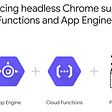# State vs Complexity

`function doSomething(value: boolean): number {  return value ? 1 : 0}`
`assert(doSomething(false).equals(correctAnswerForFalse));assert(doSomething(true).equals(correctAnswerForTrue));`
`function doSomething(a: boolean, b: boolean): number {  return (    (a ? 1 : 0) +    (b ? 1 : 0)  )}`
`┌───────┬───────┬────────┐│   a   │   b   │ assert │├───────┼───────┼────────┤│ false │ false │      0 ││ false │ true  │      1 ││ true  │ false │      1 ││ true  │ true  │      2 │└───────┴───────┴────────┘`
`let state: boolean = falsefunction doSomething(a: boolean, b: boolean): number {  const result = (    ((a || state) ? 1 : 0) +    (b ? 1 : 0)  )  state = a  return result}`
`┌───────────────┬───────────────┬─────────────────┬────────┐│ 1st time a is │ 2nd time a is │ both times b is │ assert │├───────────────┼───────────────┼─────────────────┼────────┤│ false         │ false         │ false           │      0 ││ false         │ true          │ false           │      1 ││ false         │ false         │ true            │      1 ││ true          │ false         │ false           │      1 ││ true          │ true          │ false           │      2 ││ true          │ false         │ true            │      2 ││ false         │ true          │ true            │      2 ││ true          │ true          │ true            │      3 │└───────────────┴───────────────┴─────────────────┴────────┘`
`let state: boolean = falsefunction doSomething(a: boolean, b: boolean): number {  // ...}function doSomethingElse(a: boolean, b: boolean): number {  // ...}`
`First test when doSomething(..) is called first, and then doSomethingElse(..) afterwards┌───────────────┬───────────────┬─────────────────┐│ 1st time a is │ 2nd time a is │ both times b is │├───────────────┼───────────────┼─────────────────┤│ false         │ false         │ false           ││ false         │ true          │ false           ││ false         │ false         │ true            ││ true          │ false         │ false           ││ true          │ true          │ false           ││ true          │ false         │ true            ││ false         │ true          │ true            ││ true          │ true          │ true            │└───────────────┴───────────────┴─────────────────┘Then also test when doSomethingElse(..) is called first, and then doSomething(..) afterwards┌───────────────┬───────────────┬─────────────────┐│ 1st time a is │ 2nd time a is │ both times b is │├───────────────┼───────────────┼─────────────────┤│ false         │ false         │ false           ││ false         │ true          │ false           ││ false         │ false         │ true            ││ true          │ false         │ false           ││ true          │ true          │ false           ││ true          │ false         │ true            ││ false         │ true          │ true            ││ true          │ true          │ true            │└───────────────┴───────────────┴─────────────────┘`
`┌────────┬─────────┬──────────────────┬─────────────────┐│ Params │ Total   │ Permutations     │ Permutations    ││  per   │ methods │  if stateless    │  if stateful    ││ method │ tested  │                  │                 │├────────┼─────────┼──────────────────┼─────────────────┤│      3 │       3 │              125 │ 3375            ││      3 │       4 │              125 │ 32000           ││      4 │       4 │              625 │ 160000          ││      4 │       5 │              625 │ 1953125         ││      5 │       5 │             3125 │ 145800000       ││    ... │     ... │              ... │ ...             ││      5 │      30 │             3125 │ 6.4340925e47 😱 │└────────┴─────────┴──────────────────┴─────────────────┘// ^ assuming about 30 methods per class is an average// (and there are approx 10e17 grains of sand in the whole world)`
`<input  value={firstName}  onChange={e => setFirstName(e.target.value)}/>`

--

--

--

## More from Ben Beattie-Hood

Love podcasts or audiobooks? Learn on the go with our new app.

## How to build a NestJS MVC application with YugabyteDB## Unleashing the power of React Table## Palindrome## How To Manage Sessions in Node.js Using Passport, Redis, and MySQL## XSS through base64 encoded JSON## Advantages of Developing Modern Web apps with React.js## Running End to End tests as Google Cloud Functions## Dependency Injection Vs Dependency Inversion Vs Inversion of Control, Let’s set the Record Straight## Moving into the subscription model